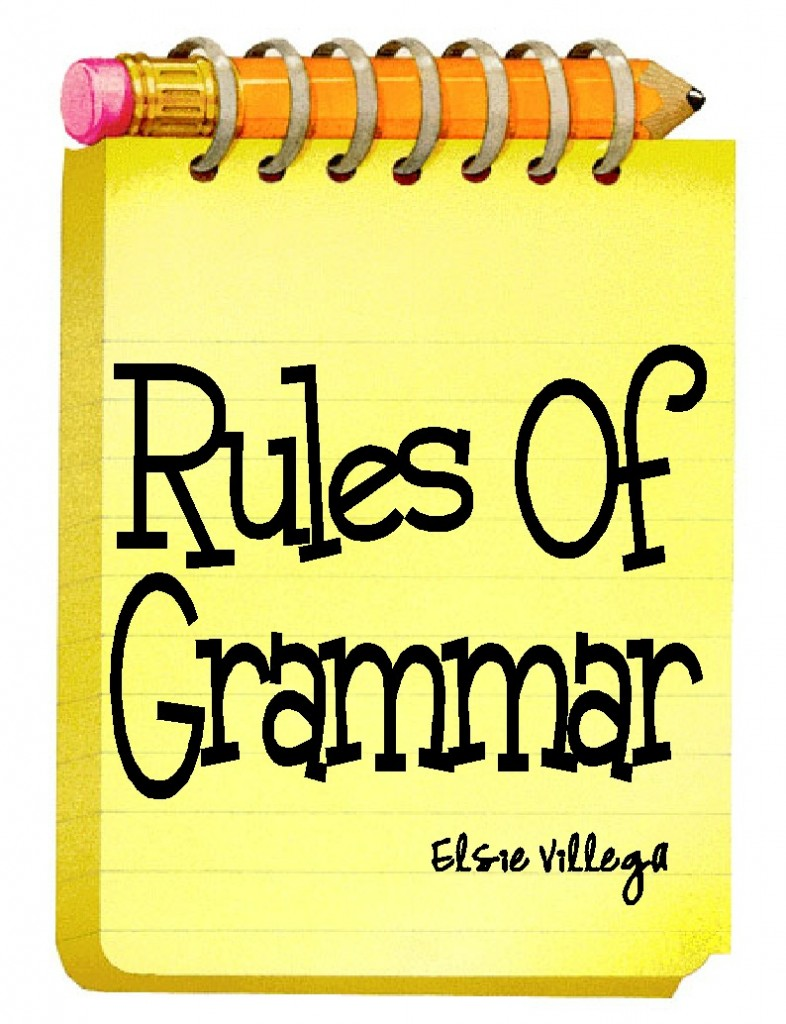December 22 2015

# Rules Of Grammar: Conjunctive Adverbs- Part 2 (Briefs Included)Conjunctive adverbs function like two different parts of speech: adverbs and coordinate conjunctions. Conjunctive adverbs are like adverbs in the meanings that they express. Conjunctive adverbs are like coordinate conjunctions in that they connect main clauses.

The meaning that is expressed by conjunctive adverbs applies to the entire clause in which it is contained.

Here are some common conjunctive adverbs along with their machine briefs:

in like manner= N-/LAOIK/MARN
in short= N-/SHORT
in the meantime= NAOEMT
later= LAIRT
likewise= LAOIKZ
meanwhile= MAOENL
moreover= MOEFR
namely= NAIM/LI
nevertheless= NEFRLS
next= NEGT
on the contrary= ONT/KRAIR
on the other hand= OERND
otherwise= OERZ
perhaps= PRAPS
so= SO
still= STIL
subsequently= SKWENLT
that is= THAS
then= THEN
therefore= THRFR
thus= THUS

December 22 2015

# Random Briefsderogatory= DROGT
retaliate= RELT or TRAELT
retaliates= RELTS or TRAELTS
retaliated= RELTD or TRAELTD
retaliating= TRAELGT
retaliation= TRAELGS
prosecute= PR-
prosecuted= PR-D
prosecutes= PR-S
prosecuting= PR-G
prosecution= PR-GS
prosecutions= PR-GSZ
prosecutor= PR-R
prosecutors= PR-RS
prosecutorial= PR-RL or PR-L
prosecutrix= PRAOUX
resilient= RILT
resilience= RILS
resiliency= RILZ or RILTS

December 21 2015

# Medical Monday: VitrectomyAccording to the Free Dictionary, a vitrectomy is: “The surgical removal of the vitreous (transparent gel that fills the eye from the iris to the retina).” The vitreous is then replaced with saline. The saline applies the same pressure that the vitreous does. This prevents the eye from collapsing.

Machine Brief:

OPTION #1: vitrectomy= VIT/REK/TO*EM
OPTION #2: vitrectomy= VIT/REK/TO*IM

December 18 2015

# Rules Of Grammar: Conjunctive Adverbs- Part 1 (Briefs Included)Conjunctive adverbs function like two different parts of speech: adverbs and coordinate conjunctions. Conjunctive adverbs are like adverbs in the meanings that they express. Conjunctive adverbs are like coordinate conjunctions in that they connect main clauses.

The meaning that is expressed by conjunctive adverbs applies to the entire clause in which it is contained.

Here are some common conjunctive adverbs along with their machine briefs:

accordingly= KWORLG
afterward= AFRD
again= GEN
also= L-S
anyhow= NI/HO*U
as a result= SRULT
at last= TLAFT
at the same time= TAIMT
besides= BESZ
consequently= KWENLT
earlier= ERL/*ER
eventually= VAENL
finally- FLAOINL
for example= FOERX
for instance= FRINS
furthermore= F*URM
hence= HENS
however= HOUFR
in any case= N-NGS
indeed= N-D
in fact= N-FT

December 18 2015

# Layout Conversion FiguresWhen measuring margins for Case CATalyst settings, a ruler that has the inches divided into tenths is a useful tool. However, such a ruler is not always readily available. Most rulers divide the inches by sixteenths. Below is a list of figures that shows the decimal equivalents for sixteenths of an inch. These conversions are the next best thing to having a ruler divided by tenths when you have to create layout settings in Case CATalyst. When needing to set various layout measurement that may not measure up to whole numbers, you can use a regular foot-long ruler and combine the whole number measurements with these decimals as need.

1/16 of an inch= .063
1/16 of an inch= .063
1/16 of an inch= .063
2/16 of an inch (or 1/8 of an inch)= .125
3/16 of an inch= .188
4/16 of an inch (or 1/4 or an inch)= .250
5/16 of an inch= .313
6/16 of an inch (or 3/8 of an inch)= .375
7/16 of an inch= .438
8/16 of an inch (or 1/2 of an inch)= .500
9/16 of an inch= .563
10/16 of an inch (or 5/8 of an inch)= .625
11/16 of an inch= .688
12/16 of an inch (or 3/4 of an inch)= .750
13/16 of an inch= .813
14/16 of an inch (or 7/8 of an inch)= .875
16/16 of an inch= 1